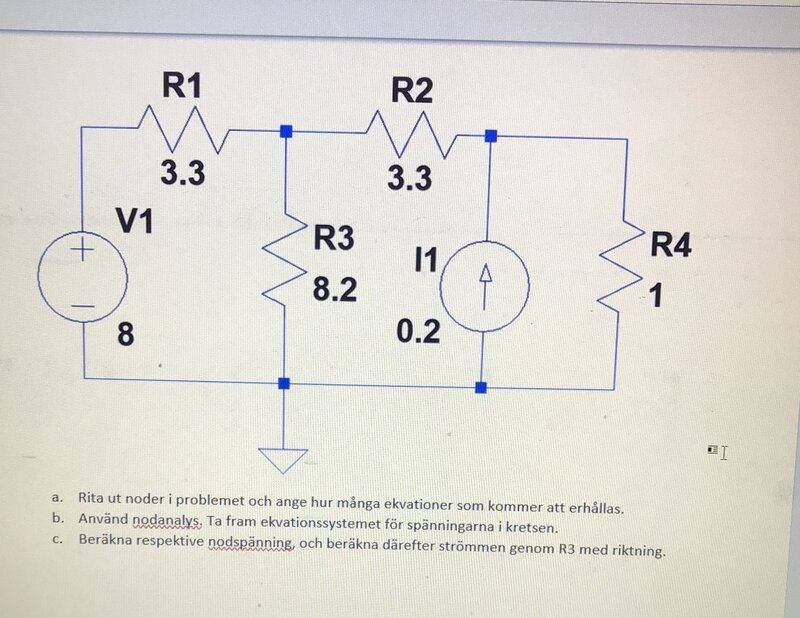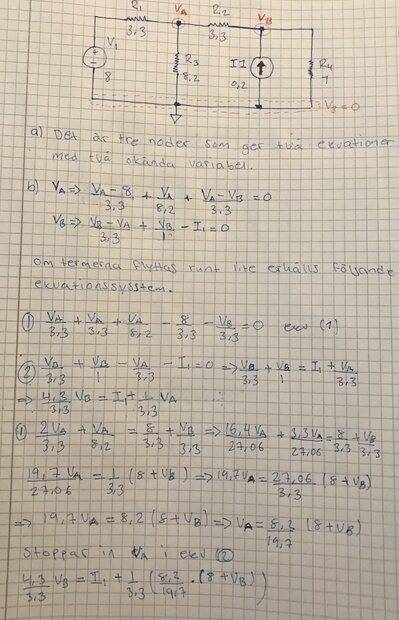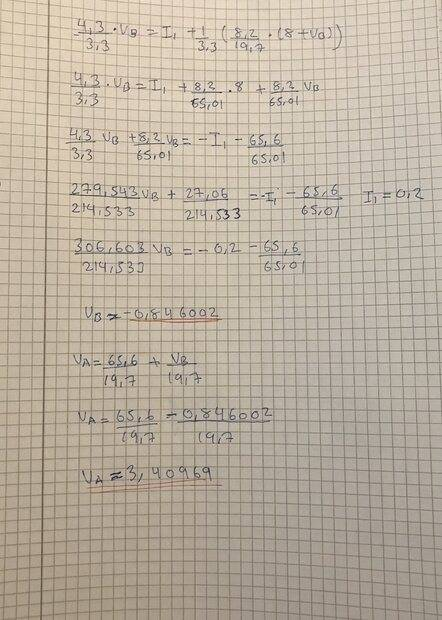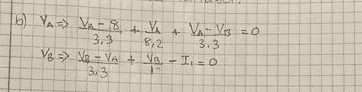# Nodal analysis of this circuit with 2 sources and 4 resistors

• Iqish
In summary, the voltages in the circuit are V_a=4.13V and V_b=1.11V. The current through R3 is I=V_a/R3.

#### Iqish

Homework Statement
nodal analysis
Relevant Equations
Someone who can help me to check if my ekvation is correct for V_a and V_b?
Here is the questiona. Plot nodes in the problem and indicate how many equations will be obtained.
b. Use node analysis. Derive the system of equations for the voltages in the circuit.question: Did I calculate right V_a and V_b?
C. Calculate the respective node voltage, and then calculate the current through R3 with direction.

#### Attachments

I only checked your initial two equations for the two unknowns, and it looks like you wrote them out correctly. Two suggestions:

** It's best to keep using the variable names as long as possible in your calculations before substituting in the values to get the final numerical solution. So in your equations, I would have used ##R_1## for as many steps as possible, instead of substituting in ##3.3## right away.

** Please learn to use LaTeX to post your math equations at PF. I would have been able to check all of your work in the time it took me to type these few words if your work were posted as LaTeX instead of as dark, small cellphone pictures. You can find a helpful LaTeX tutorial below the Edit window, "LaTeX Guide". Thanks•topsquark
In addition: If you are just trying to verify results, try the other common methods of Branch Current Analysis and\or Mesh Analysis (assuming you have learned\are learning them), its good practice.

Last edited:
•topsquark and berkeman
Hi, calculate the current through R3 with direction. Someone who can help me ?

erobz said:
In addition: If you are just trying to verify results, try the other common methods of Branch Current Analysis and\or Mesh Analysis (assuming you have learned\are learning them), its good practice.
No, not yetIqish said:
Hi, calculate the current through R3 with direction. Someone who can help me?
Have you calculated the voltage ##V_a## or not?

I don’t know how to use the LaTeX, so I inserted picture of my solution.
V_a=4.13V and V_b=1.11V

#### Attachments

Iqish said:
I don’t know how to use the LaTeX, so I inserted picture of my solution.
V_a=4.13V and V_b=1.11V
If you know ##V_a## you can find the current through ##R_3## quite readily via Ohms Law.

See the LaTeX Guide located of the bottom of any new reply

So If I use Ohms Law
I=V/R so the current through R_3 should be
I=V_a/R3
I= 4.13/8.2=0.50 A
Is that correct?

•erobz
Iqish said:
So If I use Ohms Law
I=V/R so the current through R_3 should be
I=V_a/R3
I= 4.13/8.2=0.50 A
Is that correct?
A few thoughts which might help…

##V_A## is the potential at one end of ##R_3##.
The potential at the other end of ##R_3## is zero.
So the potential difference across ##R_3## is ##V_A-0 = V_A##.

So IF your value for ##V_A## is correct, your answer for the magnitude of the current through ##R_3## is correct. (But you forgot to give the direction.)
__________

You can easily check your answers for ##V_A## and ##V_B##. Back-substitute into your initial equations. For example, for the node A equation:

##\frac {V_A-8}{3.3}+ \frac {V_A} {8.2} + \frac {V_A- V_B}{3.3} = 0##
should give
##\frac {4.13-8}{3.3}+ \frac {4.13} {8.2} + \frac{4.13- 1.1}{3.3} = 0##

Does it work (ignoring possible small rounding-errors)?
_______________

Your method of working can be shortened/simplified quite a lot.

##\frac {V_A-8}{3.3}+ \frac {V_A} {8.2} + \frac {V_A- V_B}{3.3} = 0##
Multiply both sides by ##3.3 \times 8.2##.
##8.2(V_A-8)+ 3.3(V_A) + 8.2(V_A- V_B) = 0##
Simplify this.
Do the same for the node_B equation.
You are then left with 2 (relatively) simple equations.

(And remember, as already explained by @berkeman in Post #4 [Edit: whoops, that should be Post #2], working with symbols and substituting values in the later stages, is often the most effective way of working.)
__________

You haven’t included units. You are assuming the values in the diagram have units of V, A and Ω. But they could just as easily be, for example, V, mA and kΩ. Check. Always include units.

Last edited:
•berkeman and erobz
My equation for VA is wrong,
8.2(VA−8)+3.3(VA)+8.2(VA−VB)=0
8.2(4.13-8)+3.3(4.13)+8.2(4.13-1.11)=6.659
And the same for equation for VB
1(VB-VA)+3.3VB-I1=0
(1.11-4.13)+(3.3*4.13)-0.3=0.843
I really don’t know what is wrong if the question. I don’t know how to continue this question. Someone who can help me with this one?

Iqish said:
My equation for VA is wrong,
8.2(VA−8)+3.3(VA)+8.2(VA−VB)=0
8.2(4.13-8)+3.3(4.13)+8.2(4.13-1.11)=6.659
And the same for equation for VB
1(VB-VA)+3.3VB-I1=0
(1.11-4.13)+(3.3*4.13)-0.3=0.843
I really don’t know what is wrong if the question. I don’t know how to continue this question. Someone who can help me with this one?
It looks like your two initial equations are correct - but you have made one or more mistakes in the working (algebra/arithmetic) when solving the equations.

Using back-substitution shows your solution doesn't work. This means the working is wrong - it doesn't mean the initial equations are wrong. Back substitition can not idenitify incorrect initial equations.

Try solving the equations a different way (e.g. see Post #10).

Ok I will try to solve the equation again.